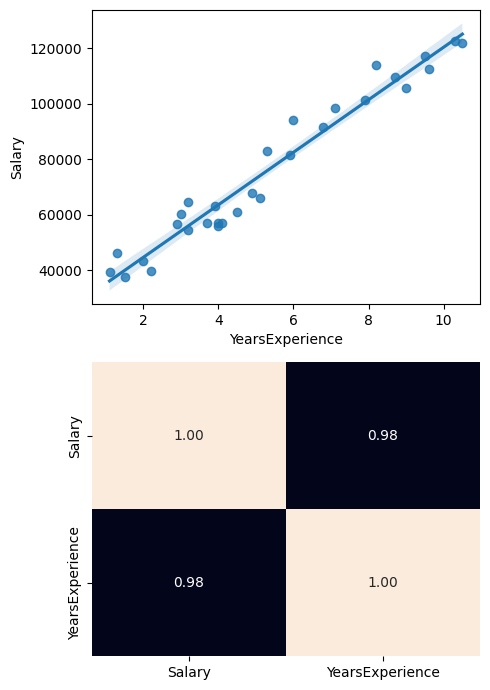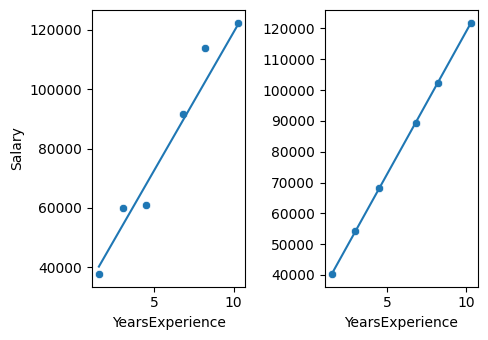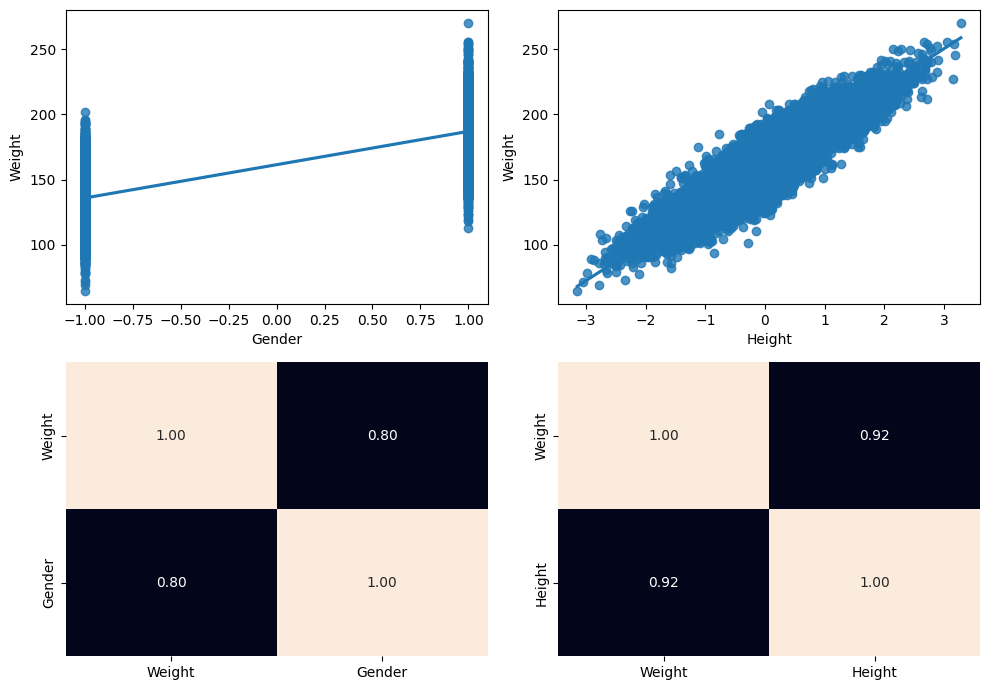Learning library

For all the self-taught geeks out there, here is our content library with most of the learning materials we have produced throughout the years.

It makes sense to start learning by reading and watching videos about fundamentals and how things work.

Data Science and Machine Learning - 16 wks

Full-Stack Software Developer - 16w

Search from all Lessons

Social & live learning

The most efficient way to learn: Join a cohort with classmates just like you, live streams, impromptu coding sessions, live tutorials with real experts, and stay motivated.

← Back to Lessons
Edit on Github
Open in Collab

# Exploring Linear Regression

## Linear Regression in Python¶

Next we will see how we can implement this model in Python. To do this, we will use the scikit-learn library.

### Simple linear regression¶

To exemplify the implementation of a simple linear regression model we will use a dataset with few instances and that has been previously treated with a full EDA.

#### Step 1. Reading the processed data set¶

In :
import pandas as pd


Out:
YearsExperienceSalary
01.139343.0
11.346205.0
22.043525.0
32.239891.0
42.956642.0

As the exploratory analysis process has not been shown in this notebook, the relationship between the predictor variable and the target variable (this is seen in the univariate analysis) will be visualized below using a dot plot:

In :
import matplotlib.pyplot as plt
import seaborn as sns

fig, axis = plt.subplots(2, 1, figsize = (5, 7))
total_data = pd.concat([train_data, test_data])

sns.regplot(ax = axis, data = total_data, x = "YearsExperience", y = "Salary")
sns.heatmap(total_data[["Salary", "YearsExperience"]].corr(), annot = True, fmt = ".2f", ax = axis, cbar = False)

plt.tight_layout()

plt.show()There is a clear linear relationship between the predictor variable and the target variable, so it can be easily modeled by this type of model. If the correlation were lower, the model would not have good accuracy.

The train set will be used to train the model, while the test set will be used to evaluate the effectiveness of the model. We will also split the predictors of the features.

Moreover, since there is only one predictor variable, it is not necessary to apply a normalization. If there were several, it would have to be applied.

In :
X_train = train_data.drop(["Salary"], axis = 1)
y_train = train_data["Salary"]
X_test = test_data.drop(["Salary"], axis = 1)
y_test = test_data["Salary"]


#### Step 2: Initialization and training of the model¶

In :
from sklearn.linear_model import LinearRegression

model = LinearRegression()
model.fit(X_train, y_train)

Out:
LinearRegression()
In a Jupyter environment, please rerun this cell to show the HTML representation or trust the notebook.

After the training process we can know the parameters (variables $a$ and $b$) that the model has fitted:

In :
print(f"Intercept (a): {model.intercept_}")
print(f"Coefficients (b): {model.coef_}")

Intercept (a): 26354.43069701219
Coefficients (b): [9277.78307971]


In this case there is only one coefficient since the linear regression is simple.

#### Step 3: Model prediction¶

In :
y_pred = model.predict(X_test)
y_pred

Out:
array([ 40271.10531658,  54187.77993614,  68104.45455571,  89443.35563904,
102432.25195063, 121915.59641802])

To compare the predicted value of the original, we can easily perform a comparative plot as follows:

In :
fig, axis = plt.subplots(1, 2, figsize = (5, 3.5))
total_data = pd.concat([train_data, test_data])

# We use the parameters adjusted in the training to draw the regression line in the plots
regression_equation = lambda x: 26354.43069701219 + 9277.78307971 * x

sns.scatterplot(ax = axis, data = test_data, x = "YearsExperience", y = "Salary")
sns.lineplot(ax = axis, x = test_data["YearsExperience"], y = regression_equation(test_data["YearsExperience"]))
sns.scatterplot(ax = axis, x = test_data["YearsExperience"], y = y_pred)
sns.lineplot(ax = axis, x = test_data["YearsExperience"], y = regression_equation(test_data["YearsExperience"])).set(ylabel = None)

plt.tight_layout()

plt.show()As we can see, the test predicted by the model will always fit the regression equation, since it is the one learned by the model. The figure on the left represents the actual values while those on the right, the predicted ones. We see that some predicted values coincide with the actual values and those that do not have a noticeable difference. We will see next the value of the metric to learn more about the performance of the algorithm.

To calculate the effectiveness of the model we will use the mean squared error (MSE) and the coefficient of determination ($R^2$), one of the most popular metrics:

In :
from sklearn.metrics import mean_squared_error, r2_score

print(f"Mean squared error: {mean_squared_error(y_test, y_pred)}")
print(f"Coefficient of determination: {r2_score(y_test, y_pred)}")

Mean squared error: 37649779.451336615
Coefficient of determination: 0.959714925174946


The lower the RMSE value, the better the model. A perfect model (a hypothetical model that can always predict the exact expected value) would have a value for this metric of 0. We observe that there is a slippage of 37 million, so we could understand that it is very bad. If we rely on the $R^2$ value, we observe that it is 95%, a very high value, then 95% of the data are explained by the model, so it is satisfactory.

#### Step 4: Optimization of results¶

This type of model cannot be optimized due to the absence of hyperparameters.

### Multiple linear regression¶

To exemplify the implementation of a simple multiple regression model we will use a data set with few instances and which has been previously treated with a full EDA.

#### Step 1. Reading the processed data set¶

In :
import pandas as pd
import matplotlib.pyplot as plt


Out:
GenderHeightWeight
0-1.0-0.575639151.275533
1-1.0-0.992843123.965162
2-1.0-0.925964124.765438
3-1.0-1.478210119.195698
41.0-1.598649146.956646

For this problem, we want to calculate the weight (weight) as a function of the height (height) and gender (gender) of the person. Therefore, weight will be the dependent variable (target variable) and height and gender, the independent variables (predictor variables). Since this is a continuous numerical prediction, we have to solve this with a multiple logistic regression model.

As the exploratory analysis process has not been shown in this notebook, the relationship between the predictor variable and the target variables (this is seen in the univariate analysis) will be visualized below using a dot plot:

In :
fig, axis = plt.subplots(2, 2, figsize = (10, 7))
total_data = pd.concat([train_data, test_data])

sns.regplot(ax = axis[0, 0], data = total_data, x = "Gender", y = "Weight")
sns.heatmap(total_data[["Weight", "Gender"]].corr(), annot = True, fmt = ".2f", ax = axis[1, 0], cbar = False)
sns.regplot(ax = axis[0, 1], data = total_data, x = "Height", y = "Weight")
sns.heatmap(total_data[["Weight", "Height"]].corr(), annot = True, fmt = ".2f", ax = axis[1, 1], cbar = False)

plt.tight_layout()

plt.show()There is a clear linear relationship between the predictor variable and the target variables, so it can be easily modeled by this type of model. If the correlation were lower, the model would not have good accuracy.

In :
X_train = train_data.drop(["Weight"], axis = 1)
y_train = train_data["Weight"]
X_test = test_data.drop(["Weight"], axis = 1)
y_test = test_data["Weight"]


#### Step 2: Initialization and training of the model¶

In :
from sklearn.linear_model import LinearRegression

model = LinearRegression()
model.fit(X_train, y_train)

Out:
LinearRegression()
In a Jupyter environment, please rerun this cell to show the HTML representation or trust the notebook.

After the training process we can know the parameters (variables $a$ and $b_1, b_2$) that the model has fitted:

In :
print(f"Intercept (a): {model.intercept_}")
print(f"Coefficients (b1, b2): {model.coef_}")

Intercept (a): 161.48606316160345
Coefficients (b1, b2): [ 9.65020608 22.88377295]


#### Step 3: Model prediction¶

In :
y_pred = model.predict(X_test)
y_pred

Out:
array([105.17851056, 188.29501423, 137.05824216, ..., 112.17172027,
130.89667195, 137.46475059])
In :
from sklearn.metrics import mean_squared_error, r2_score

print(f"Mean squared error: {mean_squared_error(y_test, y_pred)}")
print(f"Coefficient of determination: {r2_score(y_test, y_pred)}")

Mean squared error: 98.21235363443171
Coefficient of determination: 0.9075866115171992


If we rely on the value of $R^2$, we observe that it is 90%, a very high value, then 90% of the data are explained by the model, so it is satisfactory.

#### Step 4: Optimization of results¶

This type of model cannot be optimized due to the absence of hyperparameters.